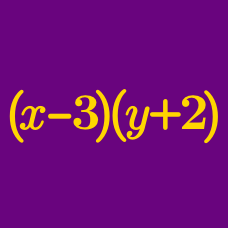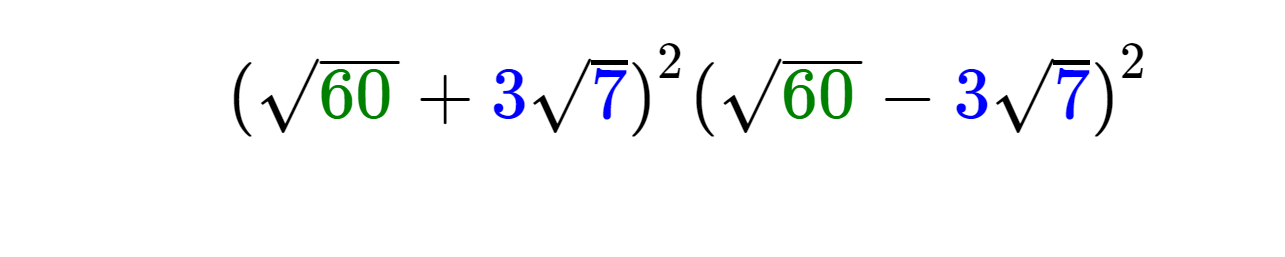Algebra

# Applying the Difference of Two Squares Identity

Given that $x^2 - y^2 = (x-y) ( x+y)$, what is the value of $31 ^2 - 19 ^2$?

Given that $a^4 - b^4 = (a^2 - b^2)(a^2 + b^2) = (a-b)(a+b)(a^2 + b^2 )$, what is the value of $5 ^4 - 4 ^4$?

If $x+y=6$, $x-y=2$ and $x^2+y^2=20$, what is the value of $x^4-y^4?$Evaluate the above expression.

Find the positive value of $N$ such that $N^2 = 169.$

×